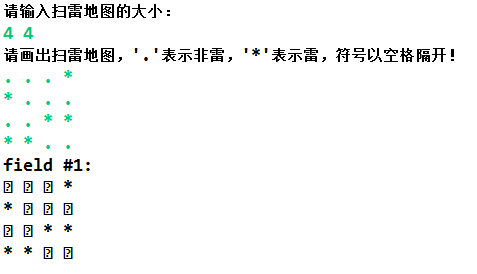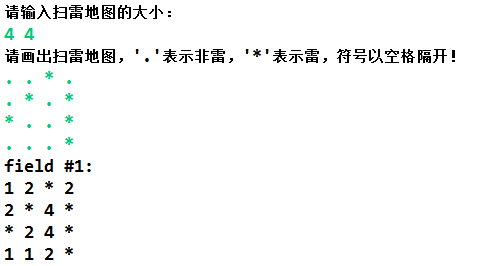# 【蓝桥备考-每周一题2】扫雷问题

package week2;
import java.util.Scanner;
public class Main {
private static final Exception MineInputException = null;
public static void main(String[] args) throws Exception {
System.out.println("请输入扫雷地图的大小：");
Scanner in = new Scanner(System.in);
int n = in.nextInt();
int m = in.nextInt();
char mine[][]=new char[n][m];
System.out.println("请画出扫雷地图，'.'表示非雷，'*'表示雷，符号以空格隔开！");
for(int i=0;i<n;i++)
for(int j=0;j<m;j++)
{
mine[i][j]=in.next().charAt(0);
}

try
{
sweeper(mine,n,m);
}
catch(MineInputException e)
{
System.out.println("捕获异常："+e.toString()+"\n");
}

System.out.println("field #1:");
for(int i=0;i<n;i++)
{
for(int j=0;j<m;j++)
{
System.out.print(mine[i][j]+" ");
}
System.out.println("");
}

}
static void sweeper(char a[][],int n,int m)throws MineInputException
{
for(int i=0;i<n;i++)
for(int j=0;j<m;j++)
{
if(a[i][j]=='*')
{
continue;
}
else if(a[i][j]=='.')
{
int count = 0;
for(int p=i-1;p<=i+1;p++)
for(int q=j-1;q<=j+1;q++)
{
if(p>=0&&q>=0&p<n&&q<m)  //查找周围雷，注意地图边界
{
if(a[p][q]=='*')
count++;
}
}
String temp=String.valueOf(count);  //int转换为char借助String实现
a[i][j]=temp.charAt(0);
}
else
{
MineInputException e = new MineInputException("输入非法，请输入'.'或'*'！");
throw e;
}
}
}
}



package week2;
public class MineInputException extends Exception{
MineInputException(String s)
{
super(s);
}
}



1.整型变量转换为字符型变量

a[i][j]=(char) count;创建一个String类型的中转变量，借助valueOf函数将整数转换为String型，然后再用String的charAt函数转换为char型

String temp=String.valueOf(count);
a[i][j]=temp.charAt(0);2.添加异常捕获块12-09149
01-18893
01-23293
02-0716万+
09-04
07-042912
11-23109
12-104450
02-08155
04-24932
03-01225
09-1041
11-1128
05-0616
11-16211
06-163934
©️2020 CSDN 皮肤主题: 大白 设计师:CSDN官方博客